The following is a discussion of how to compute image derivatives in a way that is sufficient for this course (the first assignment, in particular).
If we view an image as a function I(x,y), where the domain of I is the set of pixel locations, and the range of I is the pixel intensity, we can talk about taking its gradient. In class, we will discuss what this means for a discrete function I, which was sampled from a continuous one. In short, we want our computed derivatives of I to approximate the derivatives of the continuous function (as best we can, in the presence of sampled information). And we can do this by simply convolving the image with a derivative filter.
Suppose for now that I(x,y) is an intensity image (1 component), with range [0,1]. If it has multiple channels, we can simply average the components together to determine the intensity:
I(x,y) = (Ired(x,y) + Igreen(x,y) + Iblue(x,y)) / 3
So the gradient of the intensity image I(x,y) is: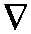I(x,y) = [ dI(x,y) / dx, dI(x,y) / dy ]

At each pixel, the gradient is a two-dimensional vector that points in the direction of maximum intensity increase (so it will tend to be perpendicular to strong edges in the image).
We can compute dI(x,y)/dx and dI(x,y)/dy by convolving I(x,y) with carefully chosen filters that approximate derivatives (known as derivative filters). Typically, these filter blur in the perpendicular direction, and find pixel differences in the other (reminiscent of numerical differentiation). Common filters for computing derivatives (used in Sobel edge detection) are:
 -1 0 1 -2 0 2 -1 0 1
Sx
 1 2 1 0 0 0 -1 -2 -1
Sy

An important note. These filters are intentionally written backwards (see the aside below for why). (For unmathematical reasons, Sy is often written upside-down because people can never seem to decide if you number images from the top going down, or the other way; so you might see Sy written upside-down in different books). Note that the direction of y chosen here is the same as images in OpenGL.
So given the discrete 2D convolution, using an (2R+1 x 2R+1) filter (where * is the convolution operation):
 x+R y+R h[x,y] = f[x,y]  *  g[x,y] =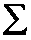f[i,j] g[x-i,y-j] i=x-R j=y-R

The above grids for g are labeled as follows:
 g[1,-1] g[0,-1] g[-1,-1] g[1, 0] g[0, 0] g[-1, 0] g[1, 1] g[0, 1] g[-1, 1]

Using these filter masks (which have to be scaled by 1/8 first, to get the appropriate scale), we can compute the image gradient as:I[x,y] = [ 1/8 I[x,y] * Sx, 1/8 I[x,y] * Sy ]

At this point, we can find the gradient magnitude in the usual way (it is a vector), and its direction of maximum increase using the inverse tangent:
direction[x,y] = arctan ( dI[x,y]/dy / dI[x,y]/dx )

Now what to do at the boundaries of the images? You might have noticed that the convolution formula (at the boundaries) requires pixel values that are outside the image. There are many ways to handle this problem (and the right way is often application dependent). We will be using one of the simplest ways (it is sort of a hack): pixels outside the image take on the value of the nearest pixel in the image. For example, I[-5,32]=I[0,32] and I[-7,-2]=I[0,0].

Aside: Why is all this stuff backwards?
Convolution seems backwards upon first inspection. Why have the filter go the other way? If you don't know what I mean, consider a discrete 1D convolution with a 3x3 filter:
 x+1 h[x] = f[x]  *  g[x] =f[i] g[x-i] i=x-1

So, if we evaluate h, we will see that the indices on f are going the opposite way from g:
 1 h =f[i] g[-i] = f[-1] g + f g + f g[-1] i=-1

So why not just negate the indices on g in the definition? It won't be backwards that way. Is this some form of torture? No. Well, not intentionally.
Basically, convolution is this way by convention. If it wasn't for this, then convolution would not be associative. With associativity, we can use the fact that if we convolve one mask with another, then the resulting combined mask can be used a mask with the same effect as separately convolving each of the original two masks (sequentially) with the image. There are deeper reasons why this is the right choice as well (relating to Convolution Theorem, which relates multiplication in the Fourier domain with convolution in the spatial domain). But that is a another story.
But... This isn't completely why the filter masks (Sx and Sy) are written down flipped. This is because when writing down filter masks, people doing image processing add a second flip, so that the filter mask is easier to look at (since you can now "directly" multiply them onto the image, without any flip at all). This is what confused me in class, because I was forgetting about this second flip.

Interpolation

Interpolation is necessary when the image is transformed geometrically and there are pixels which must have their values predicted.
Interpolation can be seen as a low pass filtering. Depending on the kind of filtering, one can get many different interpolation methods.
What follows is a case study showing the expansion by 4 in both dimensions and four interpolation methods: nearest-neighbor, linear, ideal and butterworth. In all cases the spectrum is calculated to give a better understanding of its frequency components.

Given the original image and its spectrum: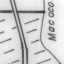---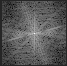Making a geometrical expansion by 4, using the equation g(4x,4y) = f(x,y), where f is the original image and g is the expanded. The other pixels of g are left as zero.
Below is the expanded image and its spectrum: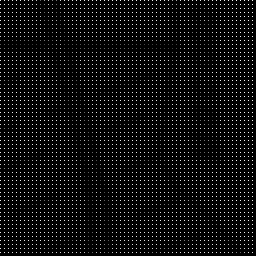---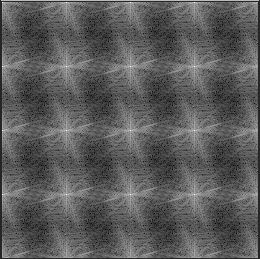We can note that the DFT of the expanded image was replicated by 4. This can be explained by recalling the DFT scaling property and remembering that the DFT is periodic.
The interpolation process must filter higher frequencies of the expanded image in order to preserve the original ones. If on the original image the minimum period possible was 2 pixels (by the Nyquist criteria), in the 4 times expanded image we should have the minimum period as 8 pixels. It is necessary to filter the image with a cutoff period of 8.
We can use four different low pass filters:

Nearest-Neighbor interpolation: average 4x4 filter:

Also called pixel replication and zero order interpolation.*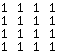=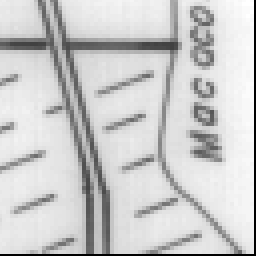---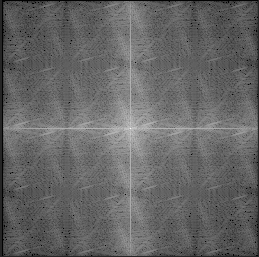.

Linear interpolation

For two dimension (image) it is called bilinear interpolation or first order interpolation.* 1/16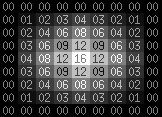=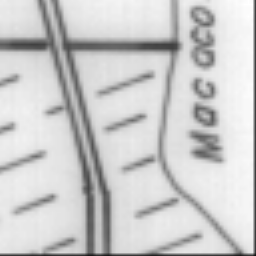---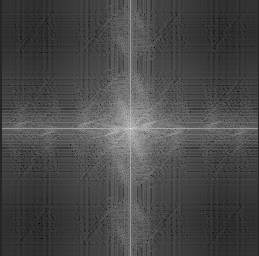Ideal interpolation

Filtering in the frequency domain using an ideal filter:x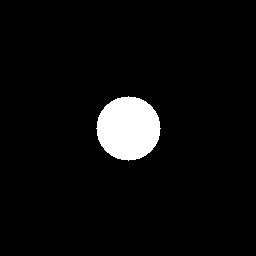=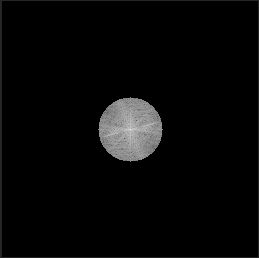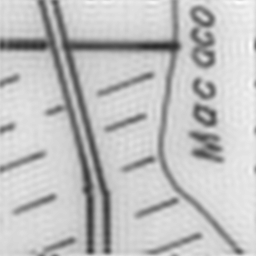Butterworth interpolation

Filtering in the frequency domain using the butterworth filter:x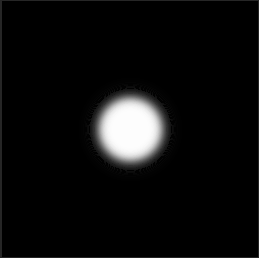=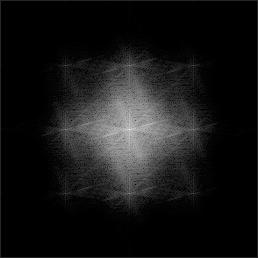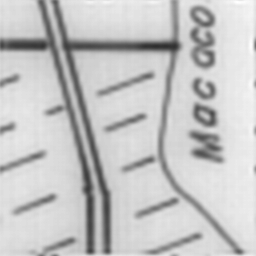Comparing the four together:---Left: Nearest Neighbor,____ Right: Bilinear---Left: Ideal,____ Right: Butterworth